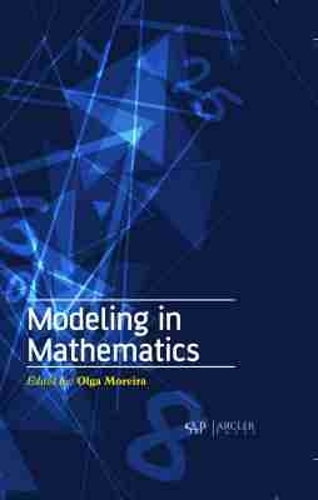•# Modeling in Mathematics (Hardback)

(editor)
£160.00
Hardback 290 Pages / Published: 30/11/2019
• We can order this

Usually dispatched within 2 weeks

Modeling in Mathematics deals with the concept of modeling in the field of mathematics. It includes a statistical and spectral model for representing noisy sounds with short-time sinusoids, robust Bayesian regularized estimation based on regression model and robust quadratic regression and its application to energy-growth consumption problem. This book also discusses about intuitionistic fuzzy weighted linear regression model with fuzzy entropy under linear restrictions, a hybrid approach of stepwise regression, logistic regression, support vector machine, and decision tree for forecasting fraudulent financial statements, dynamical analysis in explicit continuous iteration algorithm and its applications, dynamical analysis and chaos control of a discrete sis epidemic model, electricity market stochastic dynamic model and its mean stability analysis and using artificial neural networks to predict direct solar irradiation.

Publisher: Arcler Education Inc
ISBN: 9781774071434
Number of pages: 290
Dimensions: 229 x 152 mm